# ORELA Math: Circles & Arc of a Circle Chapter Exam

Exam Instructions:

Choose your answers to the questions and click 'Next' to see the next set of questions. You can skip questions if you would like and come back to them later with the yellow "Go To First Skipped Question" button. When you have completed the practice exam, a green submit button will appear. Click it to see your results. Good luck!

### Page 1

#### Question 5 5. If arc AB is 72 degrees, what is the measure of angle ACB?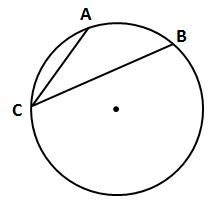### Page 2

#### Question 6 6. In the picture below, if arc CE is 172 degrees and arc BD is 80 degrees, what is the measure of angle CAE?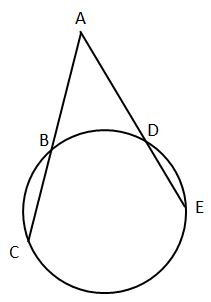#### Question 7 7. If angle OAB is 45 degrees, what is the measure of angle AOB?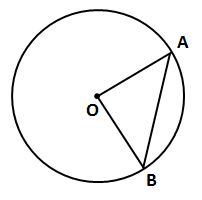#### Question 8 8. In the picture below, if arc BD is 168 degrees and arc BC is 70 degrees, what is the measure of angle BAD?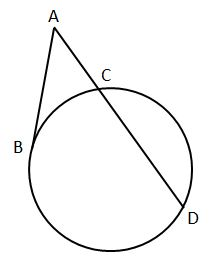#### Question 9 9. In the picture below, if arc AB is 82 degrees, what is the measure of angle BAC?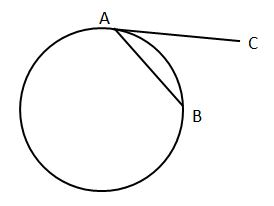#### Question 10 10. Which line is a tangent to the pictured circle?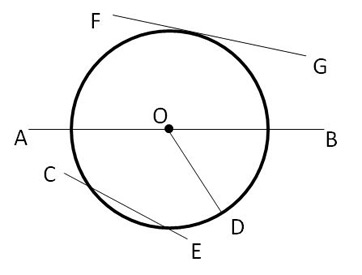### Page 3

#### Question 11 11. In the pictured circle, the measure of angle ACB is 22 degrees and the measure of angle BXD is 120 degrees. What is the measure of angle CAD?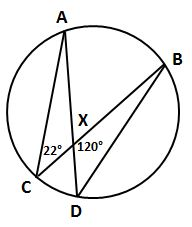#### Question 12 12. If the measure of central angle AOB is 62 degrees, what is the measure of inscribed angle ACB?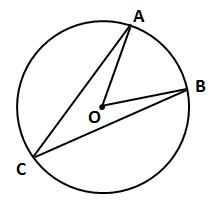#### Question 15 15. If angle AOB is 40 degrees, what is the measure of angle ACB?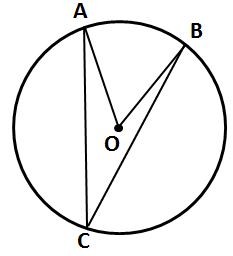### Page 4

#### Question 16 16. In the picture below, if AB is 7, BC is 19 and AD is 8, what is the length of DE?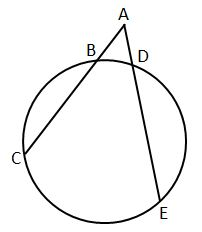#### Question 19 19. In the picture below, if AE is 8, CE is 12 and ED is 5, what is the length of EB?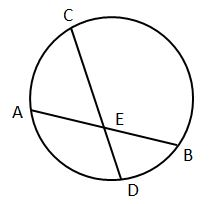#### Question 20 20. If AB and CB are tangent segments, what is the length of CB?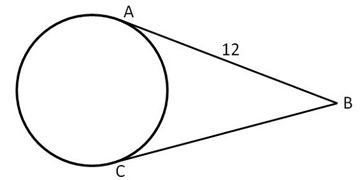### Page 6

#### Question 27 27. If angle ACB is 47 degrees, what is the measure of angle AOB?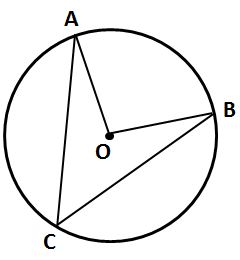#### Question 30 30. If arc AC is 106 degrees and arc BC is 82 degrees, what is the measure of angle ACB?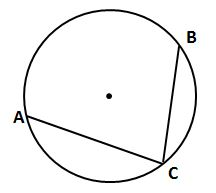#### ORELA Math: Circles & Arc of a Circle Chapter Exam Instructions

Choose your answers to the questions and click 'Next' to see the next set of questions. You can skip questions if you would like and come back to them later with the yellow "Go To First Skipped Question" button. When you have completed the practice exam, a green submit button will appear. Click it to see your results. Good luck!

Support